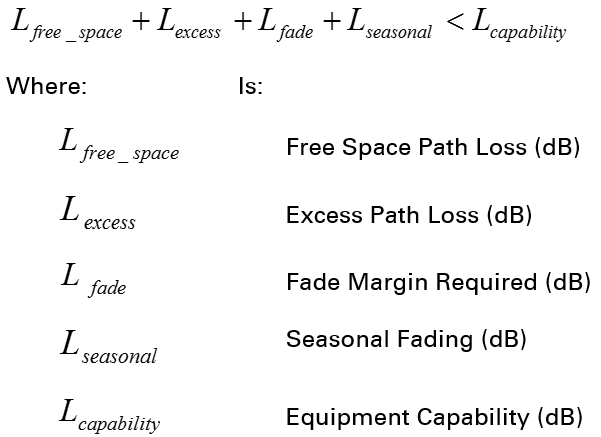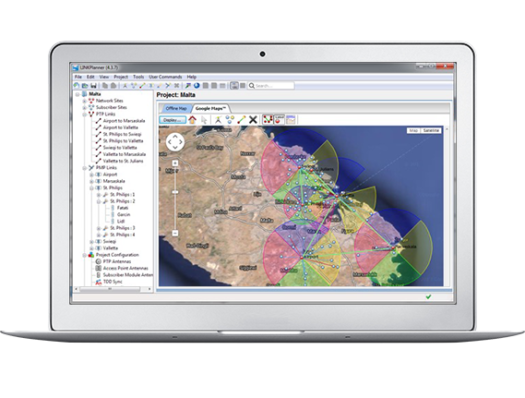# Calculating Path Loss

Path loss is the amount of attenuation the radio signal undergoes between the two ends of the link, and the calculation is used to determine whether a particular link can be installed.

The path loss is the sum of the attenuation of the path if there were no obstacles in the way (Free Space Path Loss), the attenuation caused by obstacles (Excess Path Loss) and a margin to allow for possible fading of the radio signal (Fade Margin). It also includes an allowance for the season effects of foliage growth (Seasonal Fading).

The following calculation needs to be performed to judge whether a particular link can be installed:Free space path loss is a major determinant in received (Rx) signal level.  Rx signal level, in turn, is a major factor in the system operating margin (fade margin), which is calculated as follows:

System Operating Margin (fade margin) dB = Rx signal level (dB) – Rx sensitivity (dB)

Thus, the fade margin is the difference between strength of the received signal and the strength that the receiver requires for maintaining a reliable link.Cambium Networks' LINKPlanner allows you to calculate path loss and to model “what if” scenarios – based on geography, distance, antenna height, transmit power, and other factors – to optimize system performance before purchase.

4 Likes